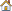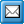﻿ Examples of Correlation Factor Calculations
QPR Knowledge Base 2017.1

Examples of Correlation Factor CalculationsMetrics User's Guide > Using QPR Metrics > Elements:

Examples of Correlation Factor CalculationsExamples of Correlation Factor CalculationsAs described on the Correlation Factor page, there are two correlation parameters: "Calculation period" and "Delay". The Calculation period is the number of periods backwards from the selected period in the element where the correlation arrow begins. The Delay sets the delay in terms of periods (i.e. months, depending on the element settings), between the start and end element of the correlation.

If the elements do not have as much periods with values as is defined in the calculation period setting, only the periods with values are used in the correlation calculation.

If there are missing values in correlation period, the values are interpolated to obtain results from correlation calculations.

In the case Latest is selected as the period, the correlation is calculated based on the latest period for which both of the measures have values in the specified series.

In the case Latest for all is selected as the period, the correlation is calculated based on the latest period for which all the measures in the scorecard have values in the specified series.

In the case Current is selected as the period, the correlation is calculated based on the current period.

All of the correlation factor calculation examples included on this page will be based on the following values:

 Period "Begin" Value "End" Value
 May 12 22 June 20 23 July 23 25 August 24 28 September 26 28

Elements "Begin" and "End" are the start and end elements of the correlation.

Example 1

Calculation period is 5 and Delay is 0. Period is either Latest,  Latest for all, or September.

All the values between May and September from both elements are used in correlation calculation. This results a correlation factor of 0,872.

Example 2

Calculation period is 5 and Delay is 1. Period is either Latest,  Latest for all, or September.

The "End" values between May and August are used from both elements as it is required to have same number of values in correlation calculations. This results in a correlation factor of 0,932.

Example 3

Calculation period is decreased to 2, Delay is 1. Period is either Latest,  Latest for all, or September.

This results in a correlation factor of 1. (Having a correlation factor of -1 or 1 is often an indication of only two values in the set).

Example 4

Calculation period is decreased to 1 and Delay is 1. Period is either Latest,  Latest for all, or September.

This produces the result N/A (not applicable). This is because a correlation factor cannot be calculated with a set of one value.

Example 5

Calculation period is 4 and Delay is 0. Period is set to August.

This results in a correlation factor of 0,822.

Example 6

Calculation period is 4, Delay is 0, and period is August. Also the value of June from "End" is removed.

This results in a correlation factor of 0,852. This is due to interpolation. When an average value of 22 and 25 (i.e. 23,5) is entered to June of the "End" element, it results in the same correlation factor.